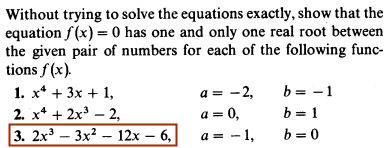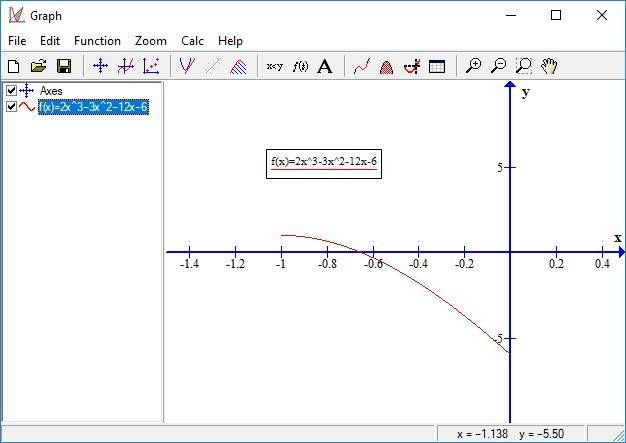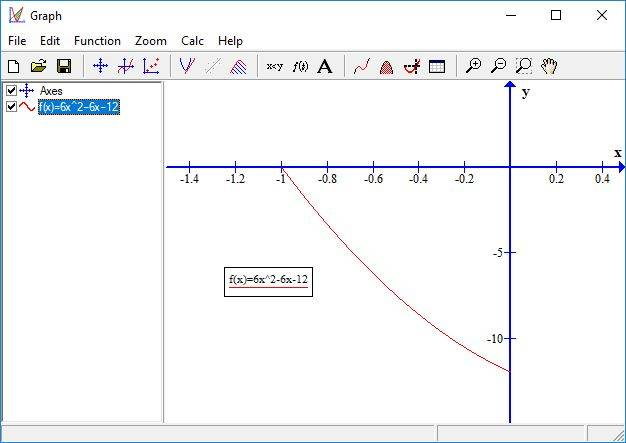# Rolle's theorem, to show there's only one root

• Karol

## Homework Statement## Homework Equations

Rolle's Theorem:
If f(a)=f(b)=0 then there is at least one a<c<b such that f'(c)=0

## The Attempt at a Solution

$$y=2x^3-3x^2-12x-6~\rightarrow~y'=6x^2-6x-12$$
The function:y':How do i know y' isn't 0 somewhere? if it's continuously descending, so i make y''=12x-6. it's a straight line, so there are no twist points in y'.
But to know y'' doesn't equal 0 somewhere i take y'''=12
Do i really need to reach y''' in order to answer the question? i think i exaggerate

#### Attachments

Last edited by a moderator:
You are trying to show there's only one real root. That is where ## f(x)=0 ## for some ## x ##. ## \\ ## The way this can be readily shown is if ## f(a)>0 ## and ##f(b) <0 ## and ## \frac{dy}{dx} < 0 ## over the entire interval. ## \\ ## Alternatively, if ## f(a)<0 ## and ## f(b)>0 ## then ## \frac{dy}{dx} > 0 ## for the entire interval will guarantee there is only one root.

## Homework Statement

View attachment 231157

## Homework Equations

Rolle's Theorem:
If f(a)=f(b)=0 then there is at least one a<c<b such that f'(c)=0

## The Attempt at a Solution

$$y=2x^3-3x^2-12x-6~\rightarrow~y'=6x^2-6x-12$$
The function:
View attachment 231158
y':
View attachment 231159
How do i know y' isn't 0 somewhere? if it's continuously descending, so i make y''=12x-6. it's a straight line, so there are no twist points in y'.
But to know y'' doesn't equal 0 somewhere i take y'''=12
Do i really need to reach y''' in order to answer the question? i think i exaggerate
Factor ##\ 6x^2 - 6x -12\ ## to find where the first derivative is zero.

Last edited by a moderator:
Y'=0 at a=(-1)
How do i prove y'≠0 on the rest of the open domain <a-b>

y' only has two zeroes, can you find the other one?

Y'=0 at a=(-1)
How do i prove y'≠0 on the rest of the open domain <a-b>
At how many points is y' = 0 for this function (in part c)? Have you investigated the intervals on which the function is increasing or decreasing?

BTW, the theorem is Rolle's Theorem. I will correct things in your thread title and elsewhere.

Y'=0 at a=(-1)
How do i prove y'≠0 on the rest of the open domain <a-b>

y'=0 at x=(-2) and x=3, between them y'<0 so y is decreasing
So between a=(-1) and b=0 y decreases
I don't need y''

y'=0 at x=(-2) and x=3
No, neither of your x values is correct. See SammyS's post #3 and show your work.
Karol said:
, between them y'<0 so y is decreasing
So between a=(-1) and b=0 y decreases
I don't need y''

$$y'=6x^2 - 6x -12$$
$$\left\{ \begin{array}{l} x_1+x_2=-\frac{b}{a}=1 \\ x_1\cdot x_2=\frac{c}{a}=(-2) \end{array}\right.$$
$$x_1=(-1),~x_2=2$$
And in between y'<0 so y is decreasing, but it doesn't matter since the theorem only demands y'≠0 in the open interval

$$y'=6x^2 - 6x -12$$
$$\left\{ \begin{array}{l} x_1+x_2=-\frac{b}{a}=1 \\ x_1\cdot x_2=\frac{c}{a}=(-2) \end{array}\right.$$
$$x_1=(-1),~x_2=2$$
And in between y'<0 so y is decreasing, but it doesn't matter since the theorem only demands y'≠0 in the open interval
Those are the correct values for which y' = 0, but why must it be that y has to be zero between x = -1 and x = 0?

Your thread title mentions Rolle's Theorem, but I don't see that this theorem is applicable in your problem. More to the point would be the Intermediate Value Theorem.

Those are the correct values for which y' = 0, but why must it be that y has to be zero between x = -1 and x = 0?

Your thread title mentions Rolle's Theorem, but I don't see that this theorem is applicable in your problem. More to the point would be the Intermediate Value Theorem.
The author of the problem was probably thinking about the not more than 1 zero part. The IVT gives you at least one zero and if you had two then Rolle's Theorem comes into play.

Yes, with the Intermediate Value Theorem:
View attachment 231302
So i even don't need to prove y decreases (by proving y'<0)
You may not " need to prove y decreases (by proving y'<0). "
However, the fact that ƒ'(x) < 0 for x ∈ [ −1, 2 ] is a reasonable way to show that ##\ f(x)=2x^3-3x^2-12x-6 \ ## decreases on that interval, so ##\ f(x)\ ## has at most one zero on that interval.

That together with the Intermediate Value Theorem is sufficient to satisfy the given problem.

You may not " need to prove y decreases (by proving y'<0). "
However, the fact that ƒ'(x) < 0 for x ∈ [ −1, 2 ] is a reasonable way to show that ##\ f(x)=2x^3-3x^2-12x-6 \ ## decreases on that interval, so ##\ f(x)\ ## has at most one zero on that interval.

That together with the Intermediate Value Theorem is sufficient to satisfy the given problem.
This is exactly what I had in mind in my previous post.

Why with the Mean Value Theorem and Rolle's Theorem there is only one root (y=0) if f(a) and f(b) have opposite signs?
The Mean Value Theorem says there is at least one root, what is the role of Rolle's theorem in that there is only one root? why does it make this if y'≠0?
Rolle's theorem is applicable if f(a)=f(b)=0, here they aren't

@Karol, you know that f(-1) > 0 and f(0) < 0, and that f is decreasing on the interval [-1, 0], and that f is continuous for all real numbers. The Mean Value Theorem guarantees that f will take on all values between f(-1) and f(0), so for some number c, f(c) must be zero. Because f is decreasing on the interval [-1, 0], it can't cross the x-axis twice, thus there can be only one zero on that interval.

Why with the Mean Value Theorem and Rolle's Theorem there is only one root (y=0) if f(a) and f(b) have opposite signs?

We are talking about this particular function on this particular interval. If f(a) and f(b) have opposite signs the IVT guarantees at least on root between a and b. There may be more.
The Mean Value Theorem says there is at least one root,

No, you mean the Intermediate Value Theorem (IVT), not the mean value theorem.

what is the role of Rolle's theorem in that there is only one root? why does it make this if y'≠0?
Rolle's theorem is applicable if f(a)=f(b)=0, here they aren't
To show this function doesn't have more than one root, there are two ways. One is to note the function is strictly decreasing so it couldn't have two roots. The other is to suppose it does have two roots, by way of contradiction. In that case Rolle's theorem would give another zero of f'(x) which gives a contradiction for this function.
[Edit:] Apparently Mark44 and I were typing at the same time.

Thank you all